# The two samples below are independent from Normal populations with equal variances. Sample 1: Sam...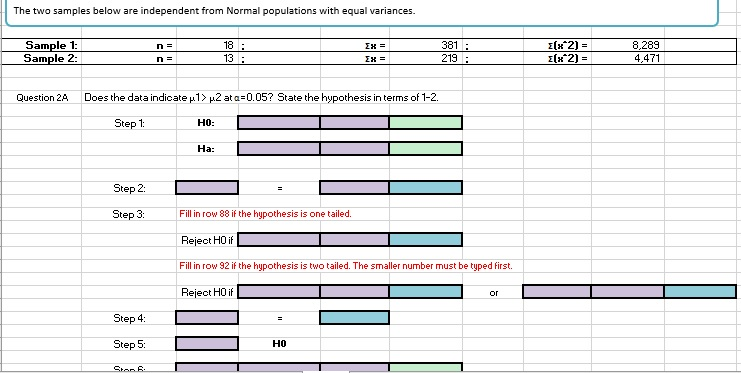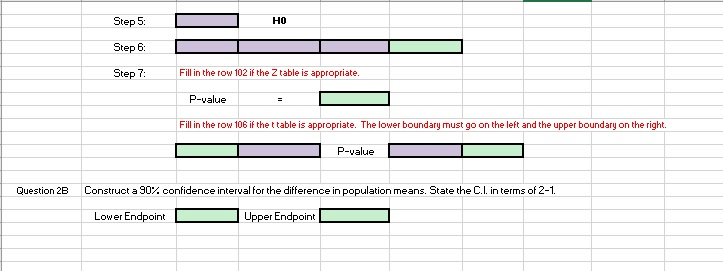The two samples below are independent from Normal populations with equal variances. Sample 1: Sample 2 18 13 381 8,289 4,471 t(x^2)= Question 2A Does the data indicate μ12μ2 at α-0.05?State the hypothesis in terms of 1-2. Step t H0: Ha: Step 2: Step 3 Fill in row 88 if the hypothesis is one tailed. Reject H0 i Fill in row 92 if the hypothesis is two tailed. The smaller number must be typed first. Reject H0 if Step 4 Step 5: HO
HO Step 6: Step 7: Fill in the row 102 if the Z table is appropriate. P-value Fill in the row 106 if the ttable is appropriate. The lower boundary must go on the left and the upper boundary on the right. P-value Question 2B Construct a 90% confidence interval for the difference in population means. State the CL interms of 2-1 Lower Endpoint Upper Endpoint

2a.
Given that,
mean(x)=381
standard deviation , s.d1=91.043
number(n1)=18
y(mean)=219
standard deviation, s.d2 =66.865
number(n2)=13
null, Ho: u1 = u2
alternate, H1: u1 > u2
level of significance, α = 0.05
from standard normal table,right tailed t α/2 =1.782
since our test is right-tailed
reject Ho, if to > 1.782
we use test statistic (t) = (x-y)/sqrt(s.d1^2/n1)+(s.d2^2/n2)
to =381-219/sqrt((8288.82785/18)+(4470.92823/13))
to =5.712
| to | =5.712
critical value
the value of |t α| with min (n1-1, n2-1) i.e 12 d.f is 1.782
we got |to| = 5.71185 & | t α | = 1.782
make decision
hence value of | to | > | t α| and here we reject Ho
p-value:right tail - Ha : ( p > 5.7119 ) = 0.00005
hence value of p0.05 > 0.00005,here we reject Ho
---------------
null, Ho: u1 = u2
alternate, H1: u1 > u2
test statistic: 5.712
critical value: 1.782
decision: reject Ho
p-value: 0.00005
we have enough evidence to support the claim that sample group1 is greater than sample group2

2b.
given that,
mean(x)=381
standard deviation , s.d1=91.043
number(n1)=18
y(mean)=219
standard deviation, s.d2 =66.865
number(n2)=13
I.
standard error = sqrt(s.d1^2/n1)+(s.d2^2/n2)
where,
sd1, sd2 = standard deviation of both
n1, n2 = sample size
standard error = sqrt((8288.828/18)+(4470.928/13))
= 28.362
II.
margin of error = t a/2 * (standard error)
where,
t a/2 = t -table value
level of significance, α = 0.1
from standard normal table, two tailed and
value of |t α| with min (n1-1, n2-1) i.e 12 d.f is 1.782
margin of error = 1.782 * 28.362
= 50.541
III.
CI = (x1-x2) ± margin of error
confidence interval = [ (381-219) ± 50.541 ]
= [111.459 , 212.541]
-----------------------------------------------------------------------------------------------
DIRECT METHOD
given that,
mean(x)=381
standard deviation , s.d1=91.043
sample size, n1=18
y(mean)=219
standard deviation, s.d2 =66.865
sample size,n2 =13
CI = x1 - x2 ± t a/2 * Sqrt ( sd1 ^2 / n1 + sd2 ^2 /n2 )
where,
x1,x2 = mean of populations
sd1,sd2 = standard deviations
n1,n2 = size of both
a = 1 - (confidence Level/100)
ta/2 = t-table value
CI = confidence interval
CI = [( 381-219) ± t a/2 * sqrt((8288.828/18)+(4470.928/13)]
= [ (162) ± t a/2 * 28.362]
= [111.459 , 212.541]
-----------------------------------------------------------------------------------------------
interpretations:
1. we are 90% sure that the interval [111.459 , 212.541] contains the true population proportion
2. If a large number of samples are collected, and a confidence interval is created
for each sample, 90% of these intervals will contains the true population proportion

##### Add Answer of: The two samples below are independent from Normal populations with equal variances. Sample 1: Sam...
Similar Homework Help Questions
• ### A study commissioned by the City of Houston in July 2013 asked a random sample of...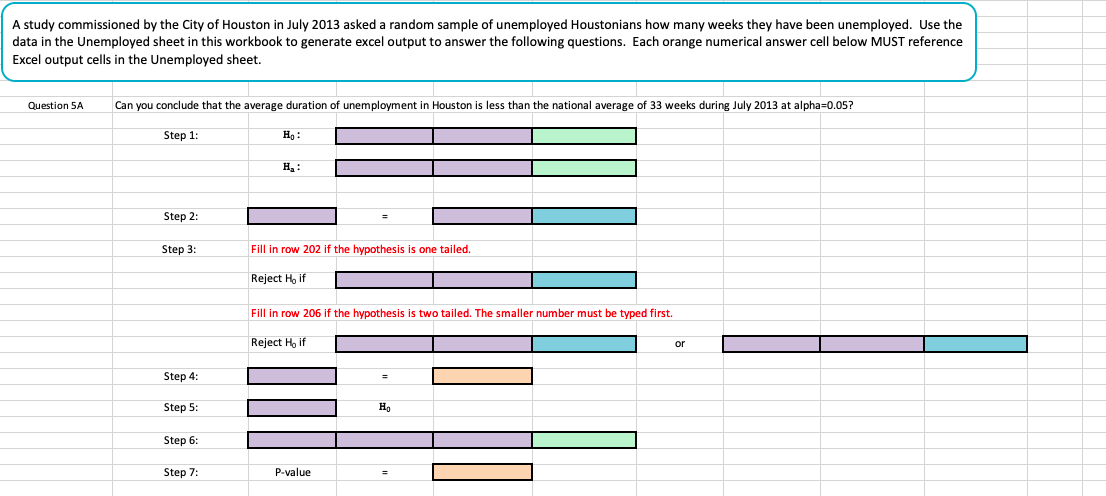A study commissioned by the City of Houston in July 2013 asked a random sample of unemployed Houstonians how many weeks they have been unemployed. Use the data in the Unemployed sheet in this workbook to generate excel output to answer the following questions. Each orange numerical answer cell below MUST reference Excel output cells in the Unemployed sheet. Question 5A Can you conclude that the average duration of unemployment in Houston is less than the national average of 33...

• ### Economists wish to look at the relationship between the price of regular unleaded gasoline in the U.S. (units-\$/gallon) and the acquisition cost of OPEC crude oil (units-\$10/barrel). Summary me...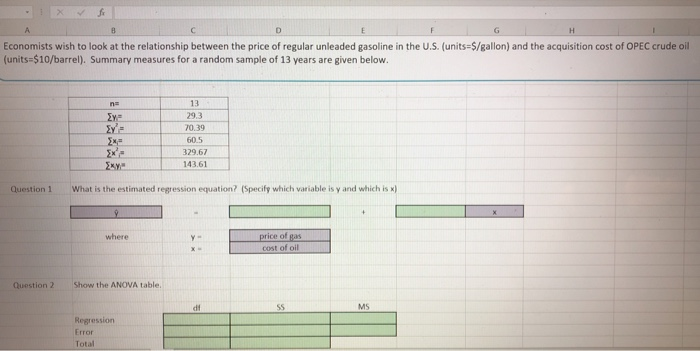Economists wish to look at the relationship between the price of regular unleaded gasoline in the U.S. (units-\$/gallon) and the acquisition cost of OPEC crude oil (units-\$10/barrel). Summary measures for a random sample of 13 years are given below 13 29.3 70.39 60.5 329.67 143.61 What is the estimated regression equation? (Specify which variable is y and which is x) Question 1 where ce of gas t of Question 2 Show the ANOVA table MS df Regression Error Total Question...

• ### (1 point) Independent random samples, each containing 90 observations, were selected from two populations. The samples...

(1 point) Independent random samples, each containing 90 observations, were selected from two populations. The samples from populations 1 and 2 produced 37 and 30 successes, respectively. Test H 0 :( p 1 − p 2 )=0 H0:(p1−p2)=0 against H a :( p 1 − p 2 )≠0 Ha:(p1−p2)≠0 . Use α=0.05 α=0.05 . (a) The test statistic is (b) The P-value is (c) The final conclusion is A. We can reject the null hypothesis that ( p 1 −...

• ### Consider the following summary statistics, calculated from two independent random samples taken from normally distributed populations....

Consider the following summary statistics, calculated from two independent random samples taken from normally distributed populations. Sample 1 Sample 2 x¯1=20.92 x¯2=26.80 s21=2.89 s22=3.81 n1=19 n2=15 Test the null hypothesis H0:μ1=μ2against the alternative hypothesis HA:μ1<μ2. a) Calculate the test statistic for the Welch Approximate t procedure. Round your response to at least 3 decimal places. b) The Welch-Satterthwaite approximation to the degrees of freedom is given by df = 27.983055. Using this information, determine the range in which the p-value...

• ### Consider the following summary statistics, calculated from two independent random samples taken from normally distributed populations....

Consider the following summary statistics, calculated from two independent random samples taken from normally distributed populations. Sample 1 Sample 2 x¯1=20.08 x¯2=24.51 s21=2.05 s22=3.20 n1=19 n2=16 Test the null hypothesis H0:μ1=μ2against the alternative hypothesis HA:μ1<μ2. a) Calculate the test statistic for the Welch Approximate t procedure. Round your response to at least 3 decimal places. b) The Welch-Satterthwaite approximation to the degrees of freedom is given by df = 28.610808. Using this information, determine the range in which the p-value...

• ### Consider the following summary statistics, calculated from two independent random samples taken from normally distributed populations....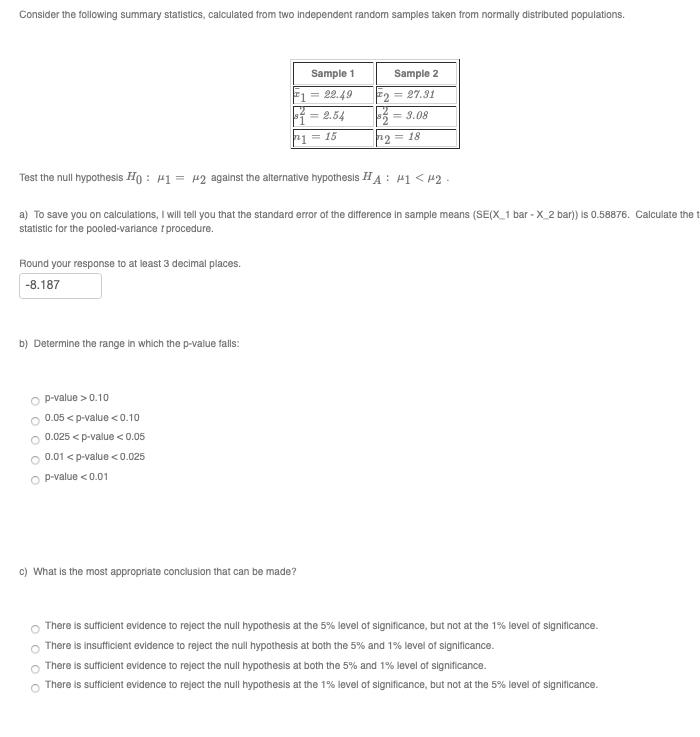Consider the following summary statistics, calculated from two independent random samples taken from normally distributed populations. Sample 1 F1 = 22.49 11 = 2.54 P1 = 15 Sample 2 F2 = 27.31 3 = 3.08 P2 = 18 Test the null hypothesis HO : H1 = 2 against the alternative hypothesis HA: MI <H2 a) To save you on calculations, I will tell you that the standard error of the difference in sample means (SE(X_1 bar - X_2 bar)) is...

• ### Independent random samples were selected from two quantitative populations, with sample sizes, means, and variances given...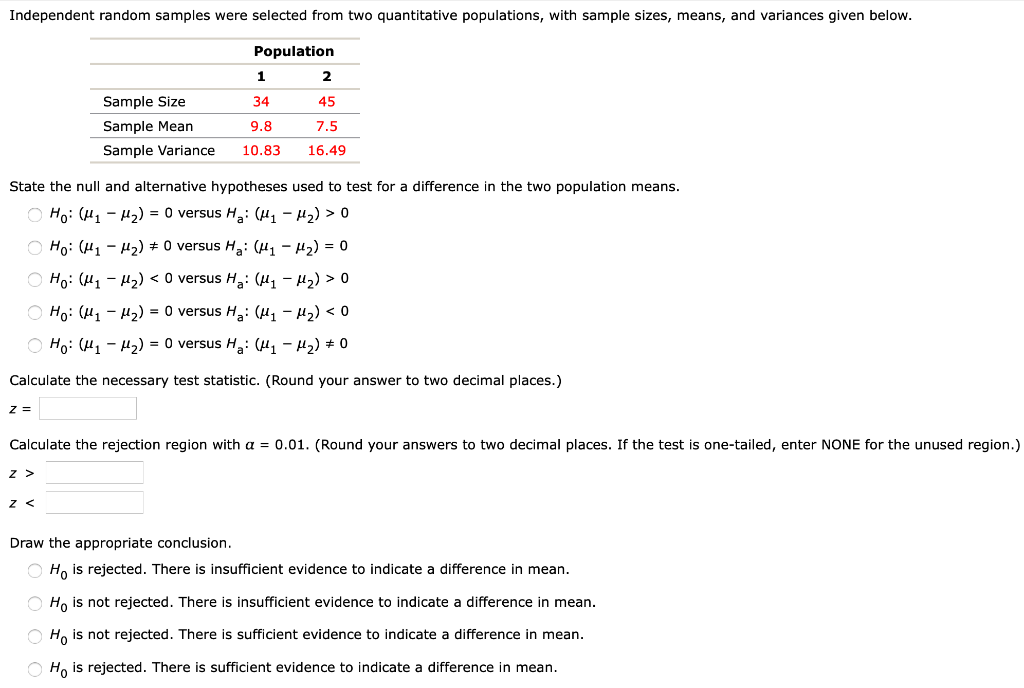Independent random samples were selected from two quantitative populations, with sample sizes, means, and variances given below. Sample Size Sample Mean Sample Variance Population 1 2 34 45 9.8 7.5 10.83 16.49 State the null and alternative hypotheses used to test for a difference in the two population means. O Ho: (41 - H2) = 0 versus Ha: (41 - M2) > 0 Ho: (41 – 12) # O versus Ha: (H1 - H2) = 0 HO: (41 – My)...

• ### (Exercise 11.1(Algorithmic)) Consider the following results for independent samples taken from two populations Sample 1 1...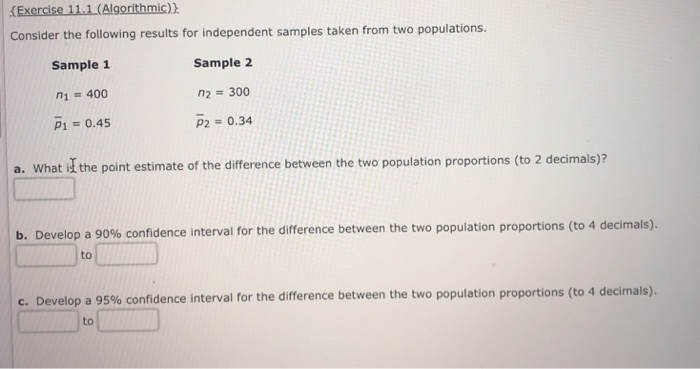(Exercise 11.1(Algorithmic)) Consider the following results for independent samples taken from two populations Sample 1 1 400 P1 0.45 Sample 2 300 p2 0.34 a. What id the point estimate of the difference between the two population proportions (to 2 decimals)i b Develop a 90% confidence interval for the difference between the two population proportions to 4 decimals to C. Develop a 95% confidence interval for the difference between the two population proportions (to 4 decimals). to Consider the hypothesis...

• ### Consider the following summary statistics, calculated from two independent random samples taken from normally distributed populations....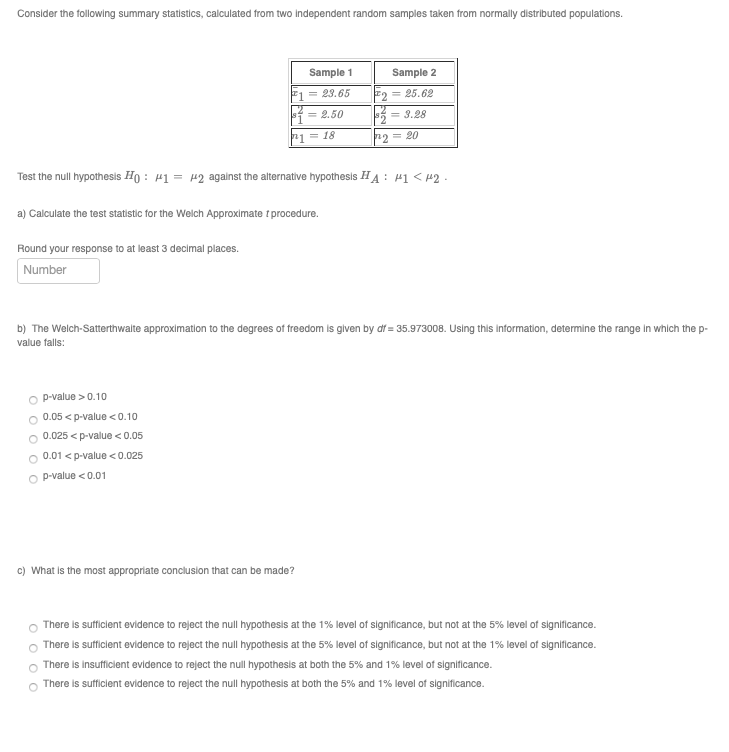Consider the following summary statistics, calculated from two independent random samples taken from normally distributed populations. Sample 1 F1 = 23.65 = 2.50 p1 = 18 Sample 2 F2 = 25.62 = 3.28 p2 = 20 Test the null hypothesis Ho: P1 = r2 against the alternative hypothesis HA : H1 CH2 a) Calculate the test statistic for the Welch Approximate procedure. Round your response to at least 3 decimal places. Number b) The Welch-Satterthwaite approximation to the degrees of...

• ### John has obtained two independent samples form two populations, where the sample statistics are shown in...

John has obtained two independent samples form two populations, where the sample statistics are shown in the table below. Assuming equal variances, he can construct a 95% confidence interval for the difference of the population means to be Sample 1 Sample 2 Mean 22.7 20.5 Variances (s^2) 5.4 3.6 Observations (sample size) 9 9 [0.08, 4.32] [1.17,5.08] [2.44,6.19] [-0.09,3.19] True or False? A new policy of “flex hours” is proposed. Random Sampling showed that 40 of 50 female workers favored...

Need Online Homework Help?